Select Page

# Feedback and Oscillators

Analog Circuits: Feedback and Oscillators

Contents

List the drawbacks of Basic Amplifier 1

Describe the Structure of a Feedback Amplifier 1

Classify the Feedback Amplifier 3

Find the Open Loop Gain and Closed Loop Gain of a Feedback Amplifier 4

Recall the Loop Gain in a Negative Feedback Amplifier 5

Discuss the Stability of Gain in case of a Negative Feedback Amplifier 6

List the Merits and Demerits of a Negative Feedback Amplifier 7

Recall the effect of Negative Feedback on the Cut-off Frequencies of an Amplifier 8

Recall the effect of Negative Feedback on Harmonic distortion on an Amplifier 9

Describe the effect of Negative Feedback on Input and Output Impedance of an Amplifier 9

Recall Current Amplifier 10

Recall Voltage Amplifier 11

Compare the Current Amplifier and the Voltage Amplifier 12

Recall the types of Feedback Topologies 14

Describe the Series-Series Feedback connection 17

Find the values of various parameters used in the Series-series Feedback Network 18

Recall the Series-Shunt Feedback Connection 19

Find the following parameters in the Series-Shunt Feedback Network: i. Input Resistance ii. Output Resistance iii. Voltage Gain iv. Feedback Ratio 20

Recall the Shunt-Series Feedback Connection 22

Find the following parameters in the Shunt-Series Feedback Network: i. Input Resistance ii. Output Resistance iii. Voltage Gain iv. Feedback Ratio 23

Recall the Shunt-Shunt Feedback Connection 24

Determine the following parameters in the Shunt-Shunt Feedback Network: i. Input Resistance ii. Output Resistance iii. Voltage Gain iv. Feedback Ratio 25

Identify the Topology of a Feedback Circuit 26

Recall the basic concept of Oscillator 27

Recall the condition for the Oscillation 28

Classify the Oscillator 29

Describe the LC Oscillator and its types 30

Describe the working of following Oscillators: i. Hartley Oscillator ii. Crystal Oscillator iii. Colpitts Oscillator iv. Clapp Oscillator 31

Recall RC Oscillator 33

Describe the following RC Phase-shift Oscillators: i. RC Phase-shift Oscillator using Op-Amp and FET 34

Describe the following RC Phase-shift Oscillators: ii. RC Phase-shift Oscillator using BJT 35

Describe Wein-bridge Oscillator 36

Describe the following Oscillators: Relaxation Oscillator, Tuned-based Oscillator, and blocking Oscillator 37

# List the drawbacks of Basic Amplifier

The basic Amplifier, which typically consists of a single amplifying device such as a transistor or vacuum tube, has several drawbacks, including:

1. Limited gain: The gain of a basic Amplifier is limited by the properties of the amplifying device and the input/output impedance of the circuit. This can make it difficult to achieve the desired level of amplification for some applications.
2. Nonlinear distortion: Basic Amplifiers can introduce nonlinear distortion, which can alter the shape of the output waveform and introduce harmonic distortion. This can be especially problematic in audio Amplifiers, where the distortion can affect the quality of the sound.
3. Temperature sensitivity: Basic Amplifiers can be sensitive to changes in temperature, which can affect their performance and stability. This is especially true for vacuum tube Amplifiers, which require a warm-up period before they can operate properly.
4. Noise: Basic Amplifiers can introduce noise into the Signal due to thermal noise, shot noise, and other sources. This noise can be especially problematic in low-level Signal applications, where it can mask the desired Signal.
5. Power consumption: Basic Amplifiers can consume a significant amount of power, which can limit their usefulness in battery-powered or low-power applications.
6. Biasing requirements: Basic Amplifiers often require careful biasing to ensure proper operation and prevent thermal runaway. This can make the circuit design more complex and increase the likelihood of errors or instability.

# Describe the Structure of a Feedback Amplifier

A Feedback Amplifier is a type of electronic Amplifier that uses Feedback to control the gain and other performance parameters of the Amplifier. The Feedback loop consists of a Network of components that sense the output Signal and provide a portion of it back to the input of the Amplifier. The Feedback Signal is subtracted from the input Signal, resulting in a reduced error Signal that drives the Amplifier output to the desired value.

The basic structure of a Feedback Amplifier consists of the following components:

1. Amplifier: The Amplifier is the main component that amplifies the input Signal to produce the output Signal. It can be any type of electronic Amplifier such as an operational Amplifier (op-amp), transistor Amplifier, or vacuum tube Amplifier.
2. Feedback Network: The Feedback Network consists of a set of components that provide a portion of the output Signal back to the input of the Amplifier. It can be a Voltage divider Network, Resistor-Capacitor (RC) Network, or any other type of Network that provides Feedback.
3. Feedback element: The Feedback element is the component that senses the output Signal and provides the Feedback Signal to the input of the Amplifier. It can be a Resistor, Capacitor, or any other type of Sensor.
4. Summing point: The summing point is the point where the input Signal and the Feedback Signal are combined. It can be an op-amp or a simple node where the two Signals are added together.

The Feedback loop can be positive or negative depending on the nature of the Signal provided by the Feedback Network. In a negative Feedback Amplifier, the Feedback Signal is subtracted from the input Signal, resulting in a reduced error Signal that drives the Amplifier output to the desired value. This type of Feedback improves the stability, linearity, and frequency response of the Amplifier. In a positive Feedback Amplifier, the Feedback Signal is added to the input Signal, resulting in an increased output Signal that drives the Amplifier to saturation. This type of Feedback is used in oscillators and other applications where a sustained output Signal is required.

Overall, the Feedback Amplifier provides a powerful tool for controlling the performance parameters of electronic circuits and systems, and is widely used in a variety of applications such as audio Amplifiers, power Amplifiers, control systems, and Signal processing circuits.

# Classify the Feedback Amplifier

Feedback Amplifiers can be classified based on several criteria, including the type of Feedback used, the Topology of the Amplifier, and the nature of the input and output Signals. Here are some common classifications:

1. Based on type of Feedback:
• Negative Feedback Amplifier: The Feedback Signal is subtracted from the input Signal, resulting in a reduced error Signal that drives the Amplifier output to the desired value. This type of Feedback improves the stability, linearity, and frequency response of the Amplifier.
• Positive Feedback Amplifier: The Feedback Signal is added to the input Signal, resulting in an increased output Signal that drives the Amplifier to saturation. This type of Feedback is used in oscillators and other applications where a sustained output Signal is required.
1. Based on Topology:
• Voltage Amplifier: The Amplifier amplifies a Voltage Signal.
• Current Amplifier: The Amplifier amplifies a Current Signal.
• Transconductance Amplifier: The Amplifier amplifies a Voltage Signal and produces a proportional output Current.
• TransResistance Amplifier: The Amplifier amplifies a Current Signal and produces a proportional output Voltage.
1. Based on input and output Signals:
• Inverting Amplifier: The output Signal is inverted with respect to the input Signal.
• Non-inverting Amplifier: The output Signal is in phase with the input Signal.
• Differential Amplifier: The Amplifier amplifies the difference between two input Signals.
• Operational Amplifier (op-amp): A high-gain Voltage Amplifier with a differential input and single-ended output.

In practice, many Feedback Amplifiers combine different types of Feedback, topologies, and input/output configurations to achieve the desired performance characteristics.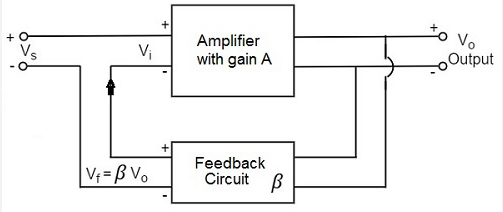# Find the Open Loop Gain and Closed Loop Gain of a Feedback Amplifier

The open-loop gain of a Feedback Amplifier is the gain of the Amplifier without any Feedback applied. It is denoted by Aol and is given by the ratio of the output Voltage to the input Voltage, when no Feedback is present.

The closed-loop gain of a Feedback Amplifier is the gain of the Amplifier with Feedback applied. It is denoted by Acl and is given by the ratio of the output Voltage to the input Voltage, when Feedback is present.

The closed-loop gain is related to the open-loop gain and the Feedback factor β by the following formula:

Acl = Aol / (1 + Aol * β)

where β is the Feedback factor, defined as the fraction of the output Signal that is fed back to the input.

To find the open-loop gain, one can measure the gain of the Amplifier at a certain frequency with no Feedback applied. This can be done by applying a small input Signal and measuring the resulting output Signal. The ratio of the output Voltage to the input Voltage is the open-loop gain.

To find the closed-loop gain, one can measure the gain of the Amplifier with Feedback applied. This can be done by connecting the Feedback Network and applying an input Signal. The output Signal is measured and the ratio of the output Voltage to the input Voltage is the closed-loop gain.

In practice, the closed-loop gain is usually preferred because it is more stable and predictable than the open-loop gain, which can vary with temperature, component variations, and other factors. The closed-loop gain can also be adjusted by changing the Feedback factor, allowing the Amplifier to be customized for different applications.

# Recall the Loop Gain in a Negative Feedback Amplifier

In a negative Feedback Amplifier, the loop gain is the gain of the Amplifier with Feedback. It is the ratio of the output Signal to the input Signal in the presence of Feedback.

The loop gain of a negative Feedback Amplifier can be expressed as:

Loop gain = Output Signal / Input Signal

The loop gain of a negative Feedback Amplifier is typically less than the open loop gain of the Amplifier, which is the gain of the Amplifier without Feedback. This is because the Feedback Signal is subtracted from the input Signal before it is amplified, which reduces the overall gain of the Amplifier.

The loop gain of a negative Feedback Amplifier is an important characteristic that determines the stability and performance of the Amplifier. A high loop gain can result in instability and oscillation, while a low loop gain can result in poor performance and a reduced Signal-to-noise ratio.

The loop gain of a negative Feedback Amplifier can be controlled by adjusting the Feedback factor, which is the ratio of the Feedback Signal to the output Signal. By adjusting the Feedback factor, it is possible to optimise the loop gain for a particular application.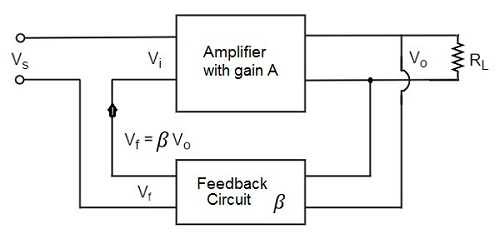# Discuss the Stability of Gain in case of a Negative Feedback Amplifier

In a negative Feedback Amplifier, the stability of the gain depends on the loop gain of the Amplifier. The loop gain is the gain of the Amplifier with Feedback, and it is the ratio of the output Signal to the input Signal in the presence of Feedback.

If the loop gain of a negative Feedback Amplifier is high, the Amplifier is prone to oscillation and instability. This is because a high loop gain can cause the Amplifier to amplify small perturbations in the Signal, resulting in oscillation.

On the other hand, if the loop gain of a negative Feedback Amplifier is low, the Amplifier is stable and the gain is well-controlled. This is because a low loop gain results in a small amplification of perturbations in the Signal, which reduces the risk of oscillation.

The loop gain of a negative Feedback Amplifier can be controlled by adjusting the Feedback factor, which is the ratio of the Feedback Signal to the output Signal. By adjusting the Feedback factor, it is possible to optimize the loop gain for a particular application and ensure the stability of the gain.

In general, negative Feedback Amplifiers are more stable than Amplifiers without Feedback because the Feedback Signal helps to stabilize the Amplifier against changes in load impedance, temperature, and other variables that can affect the Amplifier’s performance.

# List the Merits and Demerits of a Negative Feedback Amplifier

Here are some merits of a negative Feedback Amplifier:

1. Improved stability: Negative Feedback Amplifiers are generally more stable than Amplifiers without Feedback because the Feedback Signal helps to stabilize the Amplifier against changes in load impedance, temperature, and other variables that can affect the Amplifier’s performance.

2. Reduced distortion: Negative Feedback Amplifiers tend to have lower distortion than Amplifiers without Feedback because the Feedback Signal helps to cancel out nonlinearities in the Amplifier.

3. Increased bandwidth: Negative Feedback Amplifiers often have a wider bandwidth than Amplifiers without Feedback because the Feedback Signal helps to reduce the phase shift in the Amplifier.

4. Improved Signal-to-noise ratio: Negative Feedback Amplifiers often have a higher Signal-to-noise ratio than Amplifiers without Feedback because the Feedback Signal helps to reduce noise in the Amplifier.

Here are some demerits of a negative Feedback Amplifier:

1. Reduced gain: Negative Feedback Amplifiers typically have a lower gain than Amplifiers without Feedback because the Feedback Signal reduces the overall gain of the Amplifier.

2. Increased complexity: Negative Feedback Amplifiers are typically more complex than Amplifiers without Feedback because they require additional components, such as a Feedback Network, to implement the Feedback loop.

3. Increased cost: Negative Feedback Amplifiers may be more expensive to design and manufacture than Amplifiers without Feedback due to the additional components required.

4. Reduced power efficiency: Negative Feedback Amplifiers may be less power efficient than Amplifiers without Feedback because the Feedback loop consumes additional power.

Overall, the merits and demerits of a negative Feedback Amplifier depend on the specific application and the desired performance characteristics. In some cases, the improved stability, reduced distortion, and increased bandwidth of a negative Feedback Amplifier may outweigh the reduced gain and increased complexity, while in other cases, the simplicity and higher gain of an Amplifier without Feedback may be more desirable.

# Recall the effect of Negative Feedback on the Cut-off Frequencies of an Amplifier

In a negative Feedback Amplifier, the negative Feedback Signal is subtracted from the input Signal before it is amplified, which can affect the cut-off frequencies of the Amplifier. The cut-off frequencies of an Amplifier are the frequencies at which the gain of the Amplifier falls to a specified level, such as -3 dB or -20 dB.

Negative Feedback can reduce the gain of an Amplifier at high frequencies, which can increase the high-frequency cut-off frequency of the Amplifier. This is because the negative Feedback Signal is phase-inverted with respect to the input Signal and is subtracted from the input Signal before it is amplified. This can reduce the overall gain of the Amplifier at high frequencies, resulting in a higher high-frequency cut-off frequency.

On the other hand, negative Feedback can increase the gain of an Amplifier at low frequencies, which can decrease the low-frequency cut-off frequency of the Amplifier. This is because the negative Feedback Signal is subtracted from the input Signal before it is amplified, which can reduce the phase shift in the Amplifier at low frequencies. This can result in a higher gain at low frequencies and a lower low-frequency cut-off frequency.

Overall, the effect of negative Feedback on the cut-off frequencies of an Amplifier depends on the characteristics of the Amplifier and the Feedback Network. By adjusting the Feedback factor, which is the ratio of the Feedback Signal to the output Signal, it is possible to optimize the cut-off frequencies of a negative Feedback Amplifier for a particular application.

# Recall the effect of Negative Feedback on Harmonic distortion on an Amplifier

In a negative Feedback Amplifier, the negative Feedback Signal is subtracted from the input Signal before it is amplified, which can affect the harmonic distortion of the Amplifier. Harmonic distortion is the presence of harmonics, or frequencies that are integer multiples of the fundamental frequency, in the output Signal of an Amplifier.

Negative Feedback can reduce the harmonic distortion of an Amplifier by canceling out nonlinearities in the Amplifier. This is because the negative Feedback Signal is phase-inverted with respect to the input Signal and is subtracted from the input Signal before it is amplified. The negative Feedback Signal is proportional to the output Signal, which contains the harmonic components generated by the Amplifier. By subtracting the negative Feedback Signal from the input Signal, the harmonic components are canceled out, resulting in a lower level of harmonic distortion in the output Signal.

Overall, the effect of negative Feedback on the harmonic distortion of an Amplifier depends on the characteristics of the Amplifier and the Feedback Network. By adjusting the Feedback factor, which is the ratio of the Feedback Signal to the output Signal, it is possible to optimize the harmonic distortion of a negative Feedback Amplifier for a particular application. Negative Feedback Amplifiers tend to have lower harmonic distortion than Amplifiers without Feedback because the Feedback Signal helps to cancel out nonlinearities in the Amplifier.

# Describe the effect of Negative Feedback on Input and Output Impedance of an Amplifier

In a negative Feedback Amplifier, the negative Feedback Signal is subtracted from the input Signal before it is amplified, which can affect the input and output impedances of the Amplifier. The input impedance of an Amplifier is the impedance of the Amplifier at the input terminal, and the output impedance of an Amplifier is the impedance of the Amplifier at the output terminal.

Negative Feedback can increase the input impedance of an Amplifier because the Feedback Network presents a high impedance to the input Signal. This can make the Amplifier more sensitive to the input Signal and reduce the loading effect of the Amplifier on the source.

On the other hand, negative Feedback can decrease the output impedance of an Amplifier because the Feedback Network presents a low impedance to the output Signal. This can reduce the loading effect of the Amplifier on the load and improve the power transfer between the Amplifier and the load.

Overall, the effect of negative Feedback on the input and output impedances of an Amplifier depends on the characteristics of the Amplifier and the Feedback Network. By adjusting the Feedback factor, which is the ratio of the Feedback Signal to the output Signal, it is possible to optimize the input and output impedances of a negative Feedback Amplifier for a particular application.

# Recall Current Amplifier

A Current Amplifier is a type of electronic Amplifier that amplifies the Current of an input Signal rather than the Voltage. Current Amplifiers are used in a variety of applications, including instrumentation, medical devices, and telecommunications.

There are several types of Current Amplifiers, including operational Amplifiers, transconductance Amplifiers, and Current mirrors. Operational Amplifiers are widely used as Current Amplifiers because of their high gain and versatility. Transconductance Amplifiers are specialized Current Amplifiers that are used to amplify small Signals in high-precision applications. Current mirrors are simple circuits that are used to replicate the Current of an input Signal.

Current Amplifiers are typically used to amplify low-level Signals, such as those produced by Sensors or Transducers. They are often used in conjunction with other electronic components, such as Resistors, Capacitors, and Inductors, to form circuits that perform a specific function.

The performance of a Current Amplifier is typically characterized by its gain, bandwidth, and linearity. The gain of a Current Amplifier is the ratio of the output Current to the input Current, and it is typically expressed in amperes per ampere (A/A). The bandwidth of a Current Amplifier is the range of frequencies over which the Amplifier can operate, and it is typically expressed in Hertz (Hz). The linearity of a Current Amplifier is a measure of how closely the Amplifier follows a linear transfer function, and it is typically expressed as a percentage of deviation from linearity.

# Recall Voltage Amplifier

A Voltage Amplifier is a type of electronic Amplifier that amplifies the Voltage of an input Signal rather than the Current. Voltage Amplifiers are used in a variety of applications, including audio systems, power supplies, and communication systems.

There are several types of Voltage Amplifiers, including operational Amplifiers, transconductance Amplifiers, and Voltage regulators. Operational Amplifiers are widely used as Voltage Amplifiers because of their high gain and versatility. Transconductance Amplifiers are specialized Voltage Amplifiers that are used to amplify small Signals in high-precision applications. Voltage regulators are circuits that are used to maintain a constant output Voltage despite changes in the input Voltage or load conditions.

Voltage Amplifiers are typically used to amplify low-level Signals, such as those produced by Sensors or Transducers. They are often used in conjunction with other electronic components, such as Resistors, Capacitors, and Inductors, to form circuits that perform a specific function.

The performance of a Voltage Amplifier is typically characterized by its gain, bandwidth, and linearity. The gain of a Voltage Amplifier is the ratio of the output Voltage to the input Voltage, and it is typically expressed in volts per volt (V/V). The bandwidth of a Voltage Amplifier is the range of frequencies over which the Amplifier can operate, and it is typically expressed in Hertz (Hz). The linearity of a Voltage Amplifier is a measure of how closely the Amplifier follows a linear transfer function, and it is typically expressed as a percentage of deviation from linearity.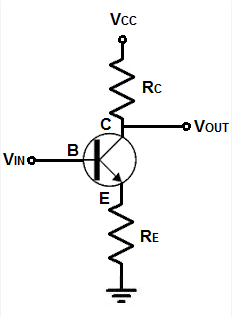# Compare the Current Amplifier and the Voltage Amplifier

Current Amplifiers and Voltage Amplifiers are two types of electronic Amplifiers that are used to amplify Signals in different ways. Here are some key differences between Current Amplifiers and Voltage Amplifiers:

The main difference between a Voltage Amplifier and a Current Amplifier is, Voltage Amplifier amplifies the Voltage whereas the Current Amplifier amplifies the Current. In other words, we can say the Voltage Amplifier provides higher Voltage gain whereas the Current Amplifier provides higher Current gain.

You can better understand the difference if you have a clear knowledge of Amplifier gain. The gain is basically the ratio of output and input. So the Voltage gain is the ratio of output Voltage and input Voltage. Similarly, the Current gain is the ratio of output Current and input Current.
So, in a Current Amplifier, the high Current gain implies that a small change in the input Current will cause a large change in the output Current. Also, a large amount of Current will flow in the output circuit when a small amount of Current flows in the input circuit.

Similarly, in a Voltage Amplifier, the high Voltage gain implies that a small change in the input Voltage will cause a large change in the output Voltage. Also, a large amount of Voltage will produce in the output when a small amount of Voltage is applied to the input. Voltage Amplifiers are mostly made up of Voltage control semiconductor devices such as Field Effect Transistors or FETs whereas Current Amplifiers are mostly made up of Current-controlled semiconductor devices such as Bipolar Junction Transistors or BJTs.
Other key differences are,

1. Voltage Amplifiers have very high impedance so they circulate low Current. Generally, the Voltage Amplifiers provide high Voltage gain and unity Current gain. On the other hand, Current Amplifiers have low impedance so they circulate high Current. So, the Current Amplifiers provide high Current gain and unity Voltage gain.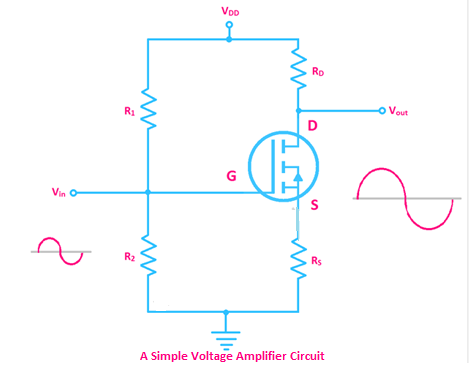2. Voltage Amplifiers produce low power loss due to their high impedance and low Current flow, on the other hand, Current Amplifiers produce high power loss due to high Current flow.

3. Voltage Amplifiers are most suitable for audio Signal amplification and driving loudspeakers whereas Current Amplifiers are most suitable for Signal processing, pre-amplification, increase the power of Transducer, etc.
4. Generally, the output and input Voltage are almost equal for a Current Amplifier but input and output Current differs. On the other hand, input and output Current is almost equal for a Voltage Amplifier but input and output Voltage differs.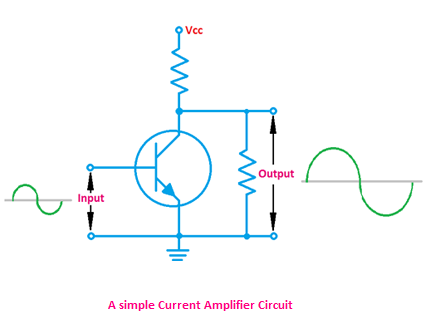5. Due to the high impedance, Capacitance effect or miller effect occurs in Voltage Amplifiers but in Current Amplifiers, Capacitance effect does not happen more.

6. Current Amplifiers are very sensitive to changes in input Current whereas Voltage Amplifiers are very sensitive to changes in Voltage.

7. Leakage Current mostly occurs in Voltage Amplifier circuits due to Capacitance effects but in Current Amplifier leakage Current happens in a very smaller amount than the normal Current.

8. When Voltage Amplifiers are used with very high-frequency Signals, noise or unwanted Signals occur but in the case of a Current Amplifier this happens in a very small amount.

# Recall the types of Feedback Topologies

There are several types of Feedback topologies that are used in electronic Amplifiers to control the gain and other performance characteristics of the Amplifier. Here are some common types of Feedback topologies:

1. Voltage Feedback: In a Voltage Feedback Amplifier, the Feedback Signal is taken from the output of the Amplifier and is compared to the input Signal. The difference between the input and output Signals is used to control the gain of the Amplifier.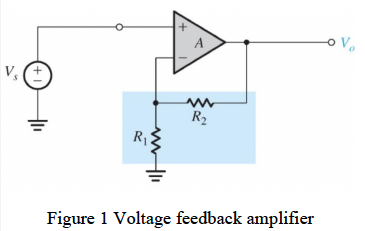2. Current Feedback: In a Current Feedback Amplifier, the Feedback Signal is taken from the output of the Amplifier and is compared to the input Signal. The difference between the input and output Signals is used to control the gain of the Amplifier.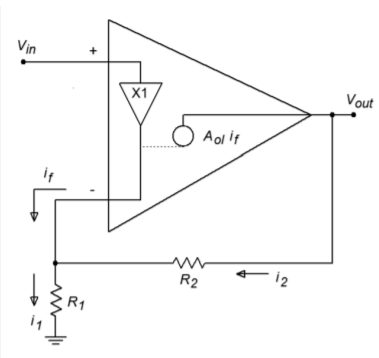3. Transconductance Feedback: In a transconductance Feedback Amplifier, the Feedback Signal is taken from the output of the Amplifier and is compared to the input Signal. The difference between the input and output Signals is used to control the gain of the Amplifier.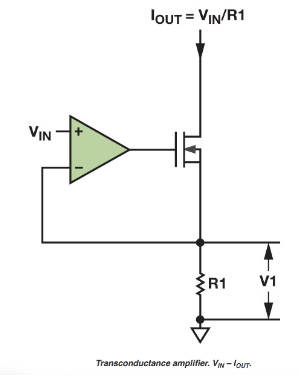4. Power Feedback: In a power Feedback Amplifier, the Feedback Signal is taken from the output of the Amplifier and is compared to the input Signal. The difference between the input and output Signals is used to control the gain of the Amplifier.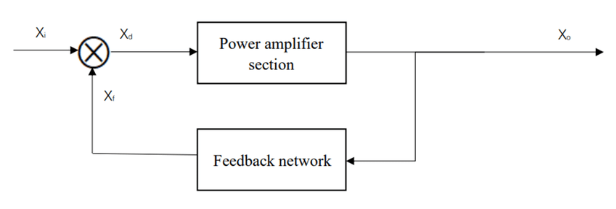5. Series Feedback: In a series Feedback Amplifier, the Feedback Signal is injected in series with the input Signal. The Feedback Signal is in phase with the input Signal and is added to the input Signal before it is amplified.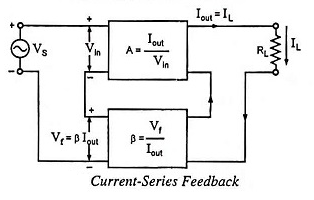6. Shunt Feedback: In a shunt Feedback Amplifier, the Feedback Signal is injected in parallel with the input Signal. The Feedback Signal is phase-inverted with respect to the input Signal and is subtracted from the input Signal before it is amplified.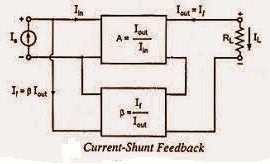The type of Feedback Topology used in an Amplifier can affect the gain, stability, bandwidth, and other performance characteristics of the Amplifier. Different Feedback topologies are suitable for different applications, and the choice of Feedback Topology depends on the specific requirements of the Amplifier.

# Describe the Series-Series Feedback connection

In a series-series Feedback connection, the Feedback Signal is taken from the output of the Amplifier and is injected in series with the input Signal. This type of Feedback connection is also known as “series negative Feedback” or “series Feedback,” as the Feedback Signal is in phase with the input Signal and is added to the input Signal before it is amplified.

In a series-series Feedback Amplifier, the Feedback Network is connected in series with the input Signal and the output Signal, as shown in the following diagram:

Input Signal -> Amplifier -> Feedback Network -> Output Signal

The Feedback Network consists of a Resistor and a Capacitor, which form a low-pass filter that passes the low-frequency components of the output Signal and attenuates the high-frequency components. The filtered output Signal is then added to the input Signal, which reduces the gain of the Amplifier at high frequencies and increases the gain at low frequencies.

Series-series Feedback Amplifiers are often used in audio applications because they have a flat frequency response and low distortion. They are also used in low-noise Amplifiers because the Feedback Network helps to reduce noise in the Amplifier.The main drawback of series-series Feedback Amplifiers is that they have a limited bandwidth due to the low-pass filter in the Feedback Network. They are not suitable for amplifying high-frequency Signals because the Feedback Network attenuates the high-frequency components of the output Signal.

# Find the values of various parameters used in the Series-series Feedback Network

In a series-series Feedback Network, the values of the Resistor and Capacitor in the Feedback Network can be chosen to control the gain and other performance characteristics of the Amplifier. Here are some steps to determine the values of the Resistor and Capacitor in a series-series Feedback Network:

1. Determine the desired gain and bandwidth of the Amplifier:

The gain and bandwidth of the Amplifier are important design parameters that determine the overall performance of the Amplifier. The gain of the Amplifier is the ratio of the output Signal to the input Signal, and it is typically expressed in decibels (dB). The bandwidth of the Amplifier is the range of frequencies over which the Amplifier can operate, and it is typically expressed in Hertz (Hz).

2. Calculate the cut-off frequency of the Feedback Network:

The cut-off frequency of the Feedback Network is the frequency at which the gain of the Amplifier falls to a specified level, such as -3 dB or -20 dB. The cut-off frequency can be calculated using the following formula:

fc = 1 / (2πRC)

where fc is the cut-off frequency in Hz, R is the Resistance in ohms, and C is the Capacitance in farads.

3. Choose the values of R and C:

The values of R and C can be chosen based on the desired gain and bandwidth of the Amplifier. If a high gain is desired, a large value of R should be chosen. If a wide bandwidth is desired, a small value of C should be chosen. It is also important to consider the power dissipation and noise performance of the Amplifier when choosing the values of R and C.

It is generally recommended to use a simulation tool, such as a SPICE simulator, to optimize the values of R and C for a particular Amplifier design. This allows you to verify the performance of the Amplifier and fine-tune the values of R and C to meet the desired specifications.

# Recall the Series-Shunt Feedback Connection

In a series-shunt Feedback connection, the Feedback Signal is taken from the output of the Amplifier and is injected in parallel with the input Signal. This type of Feedback connection is also known as “shunt negative Feedback” or “shunt Feedback,” as the Feedback Signal is phase-inverted with respect to the input Signal and is subtracted from the input Signal before it is amplified.

In a series-shunt Feedback Amplifier, the Feedback Network is connected in parallel with the input Signal and the output Signal, as shown in the following diagram:

Input Signal -> Amplifier -> Output Signal | Feedback Network

The Feedback Network consists of a Resistor and a Capacitor, which form a high-pass filter that passes the high-frequency components of the output Signal and attenuates the low-frequency components. The filtered output Signal is then subtracted from the input Signal, which reduces the gain of the Amplifier at low frequencies and increases the gain at high frequencies.

Series-shunt Feedback Amplifiers are often used in audio applications because they have a flat frequency response and low distortion. They are also used in high-frequency Amplifiers because the Feedback Network helps to reduce the phase shift in the Amplifier at high frequencies.

The main drawback of series-shunt Feedback Amplifiers is that they have a limited bandwidth due to the high-pass filter in the Feedback Network. They are not suitable for amplifying low-frequency Signals because the Feedback Network attenuates the low-frequency components of the output Signal.

# Find the following parameters in the Series-Shunt Feedback Network: i. Input Resistance ii. Output Resistance iii. Voltage Gain iv. Feedback Ratio

The Series-Shunt Feedback Network is a type of electronic circuit that is commonly used to provide negative Feedback in Amplifiers. This type of Feedback Network consists of a series element (usually a Resistor) connected in series with the input Signal, and a shunt element (usually another Resistor) connected in parallel with the output of the Amplifier. The Feedback Network is then completed by connecting the two elements together, usually with a third Resistor.

Here are the details of the parameters in the Series-Shunt Feedback Network:

i. Input Resistance:

The input Resistance of a Series-Shunt Feedback Network is the equivalent Resistance seen by the input Signal source. It can be calculated using the following formula:

Rin = Rs + (1 + Aβ)Rf

Where,

Rs = Series Resistance

Rf = Feedback Resistance

A = Open-loop gain of the Amplifier

β = Feedback ratio

ii. Output Resistance:

The output Resistance of a Series-Shunt Feedback Network is the equivalent Resistance seen by the load. It can be calculated using the following formula:

Rout = Rl//(1 + Aβ)

Where,

iii. Voltage Gain:

The Voltage gain of a Series-Shunt Feedback Network is the ratio of the output Voltage to the input Voltage. It can be calculated using the following formula:

Av = -A/(1 + Aβ)

Where,

A = Open-loop gain of the Amplifier

β = Feedback ratio

iv. Feedback Ratio:

The Feedback ratio of a Series-Shunt Feedback Network is the ratio of the Feedback element to the sum of the series and Feedback elements. It can be calculated using the following formula:

β = Rf/(Rf + Rs)

Where,

Rf = Feedback Resistance

Rs = Series Resistance

By using these formulas, we can calculate the input Resistance, output Resistance, Voltage gain, and Feedback ratio of a Series-Shunt Feedback Network. These parameters are important for analyzing the performance and stability of the Amplifier circuit.

# Recall the Shunt-Series Feedback Connection

In a shunt-series Feedback connection, the Feedback Signal is taken from the output of the Amplifier and is injected in series with the input Signal. This type of Feedback connection is also known as “series positive Feedback” or “series regenerative Feedback,” as the Feedback Signal is in phase with the input Signal and is added to the input Signal before it is amplified.

In a shunt-series Feedback Amplifier, the Feedback Network is connected in series with the input Signal and the output Signal, as shown in the following diagram:

Input Signal -> Feedback Network -> Amplifier -> Output Signal

The Feedback Network consists of a Resistor and a Capacitor, which form a low-pass filter that passes the low-frequency components of the output Signal and attenuates the high-frequency components. The filtered output Signal is then added to the input Signal, which increases the gain of the Amplifier at high frequencies and decreases the gain at low frequencies.

Shunt-series Feedback Amplifiers are often used in oscillator circuits because they can generate a sustained oscillation at a particular frequency. They are also used in high-gain Amplifiers because the Feedback Network helps to increase the gain of the Amplifier at high frequencies.

The main drawback of shunt-series Feedback Amplifiers is that they can be unstable and can produce a lot of distortion if the Feedback Network is not properly designed. They are not suitable for amplifying Signals with a wide bandwidth because the Feedback Network attenuates the high-frequency components of the output Signal.

# Find the following parameters in the Shunt-Series Feedback Network: i. Input Resistance ii. Output Resistance iii. Voltage Gain iv. Feedback Ratio

In a shunt-series Feedback Network, the values of the Resistor and Capacitor in the Feedback Network can be chosen to control the gain and other performance characteristics of the Amplifier. Here are some steps to determine the input Resistance, output Resistance, Voltage gain, and Feedback ratio in a shunt-series Feedback Network:

1. Determine the values of the Resistor and Capacitor in the Feedback Network: The values of the Resistor and Capacitor in the Feedback Network can be chosen based on the desired gain and bandwidth of the Amplifier. If a high gain is desired, a small value of the Resistor should be chosen. If a wide bandwidth is desired, a large value of the Capacitor should be chosen. It is also important to consider the power dissipation and noise performance of the Amplifier when choosing the values of the Resistor and Capacitor.

2. Calculate the input Resistance of the Amplifier: The input Resistance of the Amplifier is the Resistance of the Amplifier at the input terminal. It can be calculated using the following formula:

Rin = (1/G) || Rf

where G is the Voltage gain of the Amplifier and Rf is the Resistance of the Feedback Network.

3. Calculate the output Resistance of the Amplifier: The output Resistance of the Amplifier is the Resistance of the Amplifier at the output terminal. It can be calculated using the following formula:

Rout = R || (1/G)

where R is the Resistance of the Amplifier and G is the Voltage gain of the Amplifier.

4. Calculate the Voltage gain of the Amplifier: The Voltage gain of the Amplifier is the ratio of the output Voltage to the input Voltage. It can be calculated using the following formula:

G = (R + Rf) / R

where R is the Resistance of the Amplifier and Rf is the Resistance of the Feedback Network.

5. Calculate the Feedback ratio: Feedback ratio refers to the ratio of the amount of output Signal that is fed back to the input of an Amplifier or system, to the amount of output Signal that is not fed back. In other words, it is a measure of how much of the output Signal is used to control or modify the input Signal.

Feedback ratio is usually expressed as a fraction or a percentage. For example, if 10% of the output Signal is fed back to the input, the Feedback ratio is 0.1 or 10%.

Feedback is often used in electronic circuits to control the gain, stability, and distortion of an Amplifier or system. Negative Feedback, where the Feedback Signal is out of phase with the input Signal, can reduce the gain and distortion of an Amplifier and improve its stability. Positive Feedback, where the Feedback Signal is in phase with the input Signal, can increase the gain and distortion of an Amplifier and reduce its stability.

The Feedback ratio plays an important role in determining the behavior of a Feedback system. A higher Feedback ratio generally leads to more control and modification of the input Signal by the Feedback Signal. However, a very high Feedback ratio can lead to instability and oscillations in the system. The choice of Feedback ratio depends on the specific requirements of the system and the design goals of the circuit or Amplifier.

# Recall the Shunt-Shunt Feedback Connection

In a shunt-shunt Feedback connection, the Feedback Signal is taken from the output of the Amplifier and is injected in parallel with the input Signal. This type of Feedback connection is also known as “shunt positive Feedback” or “shunt regenerative Feedback,” as the Feedback Signal is phase-inverted with respect to the input Signal and is subtracted from the input Signal before it is amplified.

In a shunt-shunt Feedback Amplifier, the Feedback Network is connected in parallel with the input Signal and the output Signal, as shown in the following diagram:

Input Signal -> Amplifier -> Output Signal | Feedback Network

The Feedback Network consists of a Resistor and a Capacitor, which form a high-pass filter that passes the high-frequency components of the output Signal and attenuates the low-frequency components. The filtered output Signal is then subtracted from the input Signal, which increases the gain of the Amplifier at low frequencies and decreases the gain at high frequencies.

Shunt-shunt Feedback Amplifiers are often used in oscillator circuits because they can generate a sustained oscillation at a particular frequency. They are also used in high-gain Amplifiers because the Feedback Network helps to increase the gain of the Amplifier at low frequencies.

The main drawback of shunt-shunt Feedback Amplifiers is that they can be unstable and can produce a lot of distortion if the Feedback Network is not properly designed. They are not suitable for amplifying Signals with a wide bandwidth because the Feedback Network attenuates the low-frequency components of the output Signal.

# Determine the following parameters in the Shunt-Shunt Feedback Network: i. Input Resistance ii. Output Resistance iii. Voltage Gain iv. Feedback Ratio

In a shunt-shunt Feedback Network, the values of the Resistor and Capacitor in the Feedback Network can be chosen to control the gain and other performance characteristics of the Amplifier. Here are some steps to determine the input Resistance, output Resistance, Voltage gain, and Feedback ratio in a shunt-shunt Feedback Network:

1. Determine the values of the Resistor and Capacitor in the Feedback Network: The values of the Resistor and Capacitor in the Feedback Network can be chosen based on the desired gain and bandwidth of the Amplifier. If a high gain is desired, a small value of the Resistor should be chosen. If a wide bandwidth is desired, a large value of the Capacitor should be chosen. It is also important to consider the power dissipation and noise performance of the Amplifier when choosing the values of the Resistor and Capacitor.

2. Calculate the input Resistance of the Amplifier: The input Resistance of the Amplifier is the Resistance of the Amplifier at the input terminal. It can be calculated using the following formula:

Rin = R || (1/G)

where R is the Resistance of the Amplifier and G is the Voltage gain of the Amplifier.

3. Calculate the output Resistance of the Amplifier: The output Resistance of the Amplifier is the Resistance of the Amplifier at the output terminal. It can be calculated using the following formula:

Rout = R || Rf

where R is the Resistance of the Amplifier and Rf is the Resistance of the Feedback Network.

4. Calculate the Voltage gain of the Amplifier: The Voltage gain of the Amplifier is the ratio of the output Voltage to the input Voltage. It can be calculated using the following formula:

G = R / (R + Rf)

where R is the Resistance of the Amplifier and Rf is the Resistance of the Feedback Network.

5. Calculate the Feedback ratio: The Feedback ratio is the ratio of the Feedback Signal to the input Signal. It can be calculated using the following formula:

β = Rf / (R + Rf)

# Identify the Topology of a Feedback Circuit

To identify the Topology of a Feedback circuit, you can look at the connection between the input, output, and Feedback Signals. There are four possible topologies for a Feedback circuit: series-series, series-shunt, shunt-series, and shunt-shunt.

1. Series-series Feedback: In a series-series Feedback circuit, the Feedback Signal is taken from the output of the Amplifier and is injected in series with the input Signal. The Feedback Network consists of a Resistor and a Capacitor, which form a low-pass filter that passes the low-frequency components of the output Signal and attenuates the high-frequency components.

2. Series-shunt Feedback: In a series-shunt Feedback circuit, the Feedback Signal is taken from the output of the Amplifier and is injected in parallel with the input Signal. The Feedback Network consists of a Resistor and a Capacitor, which form a high-pass filter that passes the high-frequency components of the output Signal and attenuates the low-frequency components.

3. Shunt-series Feedback: In a shunt-series Feedback circuit, the Feedback Signal is taken from the output of the Amplifier and is injected in series with the input Signal. The Feedback Network consists of a Resistor and a Capacitor, which form a low-pass filter that passes the low-frequency components of the output Signal and attenuates the high-frequency components.

4. Shunt-shunt Feedback: In a shunt-shunt Feedback circuit, the Feedback Signal is taken from the output of the Amplifier and is injected in parallel with the input Signal. The Feedback Network consists of a Resistor and a Capacitor, which form a high-pass filter that passes the high-frequency components of the output Signal and attenuates the low-frequency components.

To identify the Topology of a Feedback circuit, you can examine the connection between the input, output, and Feedback Signals and determine which Feedback Network is used. You can also use a simulation tool, such as a SPICE simulator, to analyze the circuit and determine the Topology of the Feedback circuit.

# Recall the basic concept of Oscillator

An oscillator is a circuit that generates a periodic Signal, such as a sine wave or square wave. Oscillators are used in a wide variety of applications, including radio transmitters and receivers, audio generators, and timing circuits.

The basic principle of an oscillator is that it generates a continuous output Signal by using Feedback. The output Signal is fed back to the input of the Amplifier, and the Amplifier amplifies the Signal and sends it back to the output. This Feedback loop allows the oscillator to maintain a stable output Signal with a constant frequency and amplitude.

There are several types of oscillators, including relaxation oscillators, sinusoidal oscillators, and square wave oscillators. Relaxation oscillators use a simple RC (Resistor-Capacitor) circuit to generate a sawtooth or triangular waveform. Sinusoidal oscillators use a tuned LC (Inductor-Capacitor) circuit to generate a sine wave Signal. Square wave oscillators use a combination of an Amplifier and a flip-flop circuit to generate a square wave Signal.

Oscillators can be classified based on the type of Feedback used, such as positive Feedback or negative Feedback. Positive Feedback oscillators use a Feedback Network that increases the gain of the Amplifier at certain frequencies, which causes the oscillator to oscillate at those frequencies. Negative Feedback oscillators use a Feedback Network that reduces the gain of the Amplifier at certain frequencies, which causes the oscillator to oscillate at those frequencies.

# Recall the condition for the Oscillation

For an oscillator to oscillate, it must meet certain conditions. These conditions are known as the Barkhausen stability criteria. According to the Barkhausen stability criteria, an oscillator will oscillate if the following conditions are met:

1. The loop gain of the oscillator must be greater than 1: The loop gain is the product of the Amplifier gain and the Feedback factor. If the loop gain is greater than 1, the Amplifier will amplify the Feedback Signal and send it back to the output. This Feedback loop will cause the oscillator to oscillate.

2. The phase shift around the loop must be zero or an integer multiple of 360 degrees: The phase shift is the difference in phase between the input and output Signals at each stage of the Amplifier. If the phase shift around the loop is zero or an integer multiple of 360 degrees, the input and output Signals will be in phase, and the oscillator will oscillate.

3. The loop gain must be constant over the frequency range of oscillation: The loop gain must be constant over the frequency range of oscillation to ensure that the oscillator is stable and produces a constant frequency output Signal.

If these conditions are met, the oscillator will oscillate at a particular frequency, known as the resonant frequency or natural frequency. The oscillator will continue to oscillate at this frequency until the loop gain falls below 1, the phase shift around the loop becomes non-zero, or the loop gain becomes variable over the frequency range of oscillation.

# Classify the Oscillator

Oscillators can be classified based on the type of output Signal they generate, the type of Feedback used, and the type of Amplifier used. Here are some common classifications for oscillators:

1. Based on the type of output Signal:

• Sinusoidal oscillators: These oscillators generate a sine wave output Signal. They use a tuned LC (Inductor-Capacitor) circuit to produce a resonant frequency, and they can use either positive or negative Feedback.
• Square wave oscillators: These oscillators generate a square wave output Signal. They use a combination of an Amplifier and a flip-flop circuit to produce a square wave Signal. They can use either positive or negative Feedback.
• Triangle wave oscillators: These oscillators generate a triangle wave output Signal. They use a simple RC (Resistor-Capacitor) circuit to produce a triangular waveform. They can use either positive or negative Feedback.

2. Based on the type of Feedback used:

• Positive Feedback oscillators: These oscillators use a Feedback Network that increases the gain of the Amplifier at certain frequencies. This causes the oscillator to oscillate at those frequencies.
• Negative Feedback oscillators: These oscillators use a Feedback Network that reduces the gain of the Amplifier at certain frequencies. This causes the oscillator to oscillate at those frequencies.

3. Based on the type of Amplifier used:

• Transistor oscillators: These oscillators use a transistor as the Amplifier. They can generate a wide range of output frequencies and can be used in a variety of applications.
• Operational Amplifier oscillators: These oscillators use an operational Amplifier as the Amplifier. They are stable and precise, and they can generate a wide range of output frequencies.
• Crystal oscillators: These oscillators use a piezoelectric crystal as the Amplifier. They are highly stable and precise, and they can generate very high frequencies. They are often used in timing circuits and radio applications.

# Describe the LC Oscillator and its types

An LC oscillator is an oscillator that uses a tuned LC (Inductor-Capacitor) circuit to generate a sine wave output Signal. The LC circuit consists of an Inductor and a Capacitor, which are connected in parallel or in series. The resonant frequency of the LC circuit is determined by the values of the Inductor and Capacitor.

There are two main types of LC oscillators: series LC oscillators and parallel LC oscillators.

1. Series LC oscillators: In a series LC oscillator, the Inductor and Capacitor are connected in series, as shown in the following diagram:

Input -> Amplifier -> Inductor -> Capacitor -> Output

The series LC circuit acts as a band-pass filter, which allows the oscillator to oscillate at a particular frequency. The resonant frequency of the series LC circuit is given by the following formula:

f = 1 / (2π √(L*C))

where f is the resonant frequency, L is the inductance of the Inductor, and C is the Capacitance of the Capacitor.

2. Parallel LC oscillators: In a parallel LC oscillator, the Inductor and Capacitor are connected in parallel, as shown in the following diagram:

Input -> Amplifier -> Output | Inductor | Capacitor

The parallel LC circuit acts as a band-stop filter, which allows the oscillator to oscillate at a particular frequency. The resonant frequency of the parallel LC circuit is given by the following formula:

f = 1 / (2π √(C/L))

where f is the resonant frequency, L is the inductance of the Inductor, and C is the Capacitance of the Capacitor.

LC oscillators are widely used because they are simple, stable, and can generate a wide range of output frequencies. They are often used in radio transmitters and receivers, audio generators, and timing circuits.

# Describe the working of following Oscillators: i. Hartley Oscillator ii. Crystal Oscillator iii. Colpitts Oscillator iv. Clapp Oscillator

i. Hartley Oscillator:

The Hartley oscillator is a type of LC oscillator circuit that uses a tapped coil as the inductive element. The oscillator consists of an Inductor, two Capacitors, and an active device (such as a transistor or vacuum tube).

The working of the Hartley oscillator is based on the principle of LC tank circuit oscillation. The Inductor and the two Capacitors form a resonant circuit that oscillates at a particular frequency determined by the values of the components. The tapped coil provides the Feedback necessary to sustain oscillations.

When power is applied to the circuit, the active device begins to amplify the Signal from the resonant circuit. The amplified Signal is then fed back into the resonant circuit through the tapped coil. This Feedback reinforces the oscillations in the circuit, allowing it to continue oscillating at the resonant frequency.

ii. Crystal Oscillator:

A crystal oscillator is an electronic oscillator circuit that uses the mechanical resonance of a piezoelectric crystal to generate a stable frequency Signal. The oscillator circuit consists of a crystal resonator, an Amplifier, and Feedback Network.
The working of the crystal oscillator is based on the piezoelectric effect. When an alternating Voltage is applied to the crystal, it vibrates at its natural resonant frequency. This vibration generates an electrical Signal that is amplified by the Amplifier and fed back to the crystal through the Feedback Network. This Feedback sustains the oscillations at the resonant frequency of the crystal.

Crystal oscillators are known for their high accuracy and stability. They are widely used in applications where a precise and stable frequency Signal is required, such as in digital clocks, microprocessors, and communication systems.

iii. Colpitts Oscillator:

The Colpitts oscillator is another type of LC oscillator circuit that uses two Capacitors and an Inductor as the resonant elements. The oscillator circuit also includes an active device such as a transistor or vacuum tube and a Feedback Network.
The working of the Colpitts oscillator is similar to that of the Hartley oscillator. The resonant circuit, consisting of the two Capacitors and the Inductor, oscillates at a particular frequency determined by the values of the components. The active device amplifies the Signal from the resonant circuit and feeds it back to the circuit through the Feedback Network.
The Feedback Network in the Colpitts oscillator is a Voltage divider consisting of two Capacitors and a Resistor. The Voltage across the Capacitor in the Voltage divider provides the necessary Feedback to sustain oscillations in the circuit.

iv. Clapp Oscillator:

The Clapp oscillator is a modification of the Colpitts oscillator that uses an additional Capacitor in the resonant circuit. The additional Capacitor provides a more stable frequency output than the Colpitts oscillator.

The working of the Clapp oscillator is similar to that of the Colpitts oscillator. The resonant circuit, consisting of the three Capacitors and the Inductor, oscillates at a particular frequency determined by the values of the components. The active device amplifies the Signal from the resonant circuit and feeds it back to the circuit through the Feedback Network.
The Feedback Network in the Clapp oscillator is also a Voltage divider consisting of two Capacitors and a Resistor, but one of the Capacitors is now in the resonant circuit. This additional Capacitor provides a more stable frequency output than the Colpitts oscillator. The Clapp oscillator is commonly used in radio frequency (RF) applications where a stable frequency Signal is required.

# Recall RC Oscillator

An RC oscillator is an oscillator that uses a simple RC (Resistor-Capacitor) circuit to generate a periodic output Signal, such as a sawtooth or triangular waveform. RC oscillators are also known as relaxation oscillators because they rely on the charging and discharging of a Capacitor to produce an oscillating output Signal.

The RC oscillator circuit is shown in the following diagram:

Input -> Amplifier -> Output | Resistor | Capacitor

The RC oscillator uses positive Feedback to generate an oscillating output Signal. The Feedback Network consists of a Resistor and a Capacitor, which form a low-pass filter. The filtered output Signal is then fed back to the input of the Amplifier.

As the Capacitor charges and discharges, the output Signal of the Amplifier changes, and the oscillator generates a periodic output Signal. The frequency of the oscillator is determined by the time constant of the RC circuit, which is given by the following formula:

t = RC

where t is the time constant, R is the Resistance of the Resistor, and C is the Capacitance of the Capacitor. The oscillation frequency is given by the following formula:

f = 1 / (2π √(R*C))

RC oscillators are simple and inexpensive, and they can generate a wide range o.3.3-2f frequencies. They are often used in timing circuits and pulse generation applications.

# Describe the following RC Phase-shift Oscillators: i. RC Phase-shift Oscillator using Op-Amp and FET

1. RC phase-shift oscillator using an op-amp:

An RC phase-shift oscillator using an op-amp is an oscillator that uses an operational Amplifier and an RC Network to generate a sine wave output Signal. The RC phase-shift oscillator circuit is shown in the following diagram:

Input -> Op-amp -> Output | Resistor | Capacitor

The RC phase-shift oscillator uses negative Feedback to generate an oscillating output Signal. The Feedback Network consists of three Resistors and three Capacitors, which form a low-pass filter. The filtered output Signal is then fed back to the input of the op-amp through a phase shift Network, which consists of two additional Resistors and two additional Capacitors.

The phase shift Network introduces a phase shift of 180 degrees at the resonant frequency of the oscillator. This phase shift, combined with the negative Feedback, causes the oscillator to oscillate at the resonant frequency.

The resonant frequency of the RC phase-shift oscillator is given by the following formula:

f = 1 / (2π √(R1*C1))

where f is the resonant frequency, R1 is the Resistance of the first Resistor in the phase shift Network, and C1 is the Capacitance of the first Capacitor in the phase shift Network.

2. RC phase-shift oscillator using a FET:

The RC phase-shift oscillator is a type of electronic oscillator that uses RC circuits (Resistor-Capacitor circuits) to generate a sinusoidal waveform. It is typically used in audio applications such as tone generation and audio synthesis.

To build an RC phase-shift oscillator using a FET (field-effect transistor), you can follow these steps:

1. Choose a suitable FET. The FET should have a high input impedance and low output impedance. Popular choices include the JFET (junction field-effect transistor) and MOSFET (metal-oxide-semiconductor field-effect transistor).
2. Design the RC Network. The RC Network consists of several stages of RC circuits, typically three or four stages. The values of the Resistors and Capacitors are chosen such that the total phase shift around the loop is 360 degrees at the desired frequency of oscillation. For example, if you want to generate a 1 kHz tone, each stage of the RC Network should provide a phase shift of 60 degrees.
3. Connect the FET. The FET is used as an Amplifier to provide the necessary gain to sustain oscillations. The FET is connected in a common source configuration, with the input Signal applied to the gate and the output taken from the drain.
4. Add Feedback. A small portion of the output Signal is fed back to the input through a Capacitor. This Feedback Signal reinforces the input Signal and helps to sustain oscillations.
5. Bias the FET. The FET must be biased to operate in its linear region. This is usually accomplished using a Resistor connected between the gate and the negative power supply, and another Resistor connected between the drain and the positive power supply.
6. Tune the circuit. The frequency of oscillation can be adjusted by varying the values of the Resistors and Capacitors in the RC Network. A frequency counter or an oscilloscope can be used to measure the output frequency.

Overall, the RC phase-shift oscillator using a FET is a simple and effective circuit for generating sinusoidal waveforms. However, it may have some limitations in terms of frequency stability and waveform distortion, especially at high frequencies.

# Describe the following RC Phase-shift Oscillators: ii. RC Phase-shift Oscillator using BJT

An RC phase-shift oscillator using a BJT (bipolar junction transistor) is an oscillator that uses a bipolar junction transistor and an RC Network to generate a sine wave output Signal. The RC phase-shift oscillator circuit is shown in the following diagram:

Input -> BJT -> Output | Resistor | Capacitor

The RC phase-shift oscillator uses negative Feedback to generate an oscillating output Signal. The Feedback Network consists of three Resistors and three Capacitors, which form a low-pass filter. The filtered output Signal is then fed back to the input of the BJT through a phase shift Network, which consists of two additional Resistors and two additional Capacitors.

The phase shift Network introduces a phase shift of 180 degrees at the resonant frequency of the oscillator. This phase shift, combined with the negative Feedback, causes the oscillator to oscillate at the resonant frequency.

The resonant frequency of the RC phase-shift oscillator is given by the following formula:

f = 1 / (2π √(R1*C1))

where f is the resonant frequency, R1 is the Resistance of the first Resistor in the phase shift Network, and C1 is the Capacitance of the first Capacitor in the phase shift Network.

RC phase-shift oscillators using BJTs are simple and inexpensive, and they can generate a wide range of frequencies. They are often used in timing circuits and pulse generation applications.

# Describe Wein-bridge Oscillator

A Wein-bridge oscillator is an oscillator that uses a balanced Wein-bridge circuit to generate a sine wave output Signal. The Wein-bridge oscillator circuit is shown in the following diagram:

Input -> Amplifier -> Output | Resistor | Capacitor

The Wein-bridge oscillator uses negative Feedback to generate an oscillating output Signal. The Feedback Network consists of a Resistor and a Capacitor, which form a low-pass filter. The filtered output Signal is then fed back to the input of the Amplifier through a phase shift Network, which consists of an additional Resistor and an additional Capacitor.

The phase shift Network introduces a phase shift of 180 degrees at the resonant frequency of the oscillator. This phase shift, combined with the negative Feedback, causes the oscillator to oscillate at the resonant frequency.

The resonant frequency of the Wein-bridge oscillator is given by the following formula:

f = 1 / (2π √(R*C))

where f is the resonant frequency, R is the Resistance of the Resistor in the phase shift Network, and C is the Capacitance of the Capacitor in the phase shift Network.

Wein-bridge oscillators are stable and precise, and they can generate a wide range of frequencies. They are often used in audio frequency applications and Signal generators.

# Describe the following Oscillators: Relaxation Oscillator, Tuned-based Oscillator, and blocking Oscillator

1. Relaxation oscillator:

A relaxation oscillator is an oscillator that uses a simple RC (Resistor-Capacitor) circuit to generate a periodic output Signal, such as a sawtooth or triangular waveform. RC oscillators are also known as relaxation oscillators because they rely on the charging and discharging of a Capacitor to produce an oscillating output Signal.

The relaxation oscillator circuit is shown in the following diagram:

Input -> Amplifier -> Output | Resistor | Capacitor

The relaxation oscillator uses positive Feedback to generate an oscillating output Signal. The Feedback Network consists of a Resistor and a Capacitor, which form a low-pass filter. The filtered output Signal is then fed back to the input of the Amplifier.

As the Capacitor charges and discharges, the output Signal of the Amplifier changes, and the oscillator generates a periodic output Signal. The frequency of the oscillator is determined by the time constant of the RC circuit, which is given by the following formula:

t = RC

where t is the time constant, R is the Resistance of the Resistor, and C is the Capacitance of the Capacitor. The oscillation frequency is given by the following formula:

f = 1 / (2π √(R*C))

Relaxation oscillators are simple and inexpensive, and they can generate a wide range of frequencies. They are often used in timing circuits and pulse generation applications.

2. Tuned-based oscillator:

A tuned-based oscillator is an oscillator that uses a resonant circuit, such as an LC (Inductor-Capacitor) or RC (Resistor-Capacitor) circuit, to generate an oscillating output Signal. The resonant circuit is usually tuned to a particular frequency, and the oscillator generates an output Signal at that frequency.

Tuned-based oscillators can be classified into two main types: LC oscillators and RC oscillators.

• LC oscillators: LC oscillators use an LC circuit to generate an oscillating output Signal. There are two main types of LC oscillators: series LC oscillators and parallel LC oscillators.
• RC oscillators: RC oscillators use an RC circuit to generate a periodic output Signal, such as a sawtooth or triangular waveform. RC oscillators are also known as relaxation oscillators because they rely on the charging and discharging of a Capacitor to produce an oscillating output Signal.

Tuned-based oscillators are stable and precise, and they can generate a wide range of frequencies. They are often used in radio transmitters and receivers, audio generators, and timing circuits.

3. Blocking oscillator:

A blocking oscillator is an oscillator that uses a resonant circuit, such as an LC (Inductor-Capacitor) or RC (Resistor-Capacitor) circuit, to generate an oscillating output Signal. The resonant circuit is usually tuned to a particular frequency, and the oscillator generates an output Signal at that frequency.

The blocking oscillator circuit is shown in the following diagram:

Input -> Amplifier -> Output | Resistor | Capacitor

The blocking oscillator uses negative Feedback to generate an oscillating output Signal. The Feedback Network consists of a Resistor and a Capacitor, which form a low-pass filter. The filtered output Signal is then fed back to the input of the Amplifier through a blocking diode, which prevents the Feedback Signal from being passed to the input.